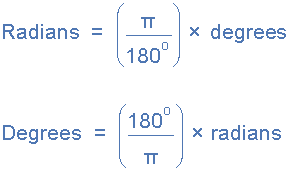# Numpy Universal Functions

Last updated: April 9th, 2019# Numpy Universal functions (ufuncs)¶

In this lesson we'll learn about numpy "Universal Functions", or ufuncs. Ufuncs are functions that operate on arrays in an element-by-element basis, in a really efficient way.

In our previous lecture we introduced Vectorized Operations, which in turn, are one example of ufuncs. The important trait about ufuncs is that they're optimized internally using C code, which makes them REALLY fast and efficient.

We'll start by comparing the efficiency of regular "python loops" vs numpy vectorized operations (ufuncs).## Hands on!¶

Let's start with an example: given an array of numbers, we want to compare the reciprocal of each element. Our first approach will be with a regular Python for-loop (example taken from Data Science Handbook):

In [ ]:
import numpy as np

In [ ]:
def compute_reciprocals(values):
output = np.empty(len(values))
for i in range(len(values)):
output[i] = 1.0 / values[i]
return output

In [ ]:
values = np.random.randint(1, 999, size=10)
values

In [ ]:
compute_reciprocals(values)


The numpy, vectorized operation counterpart is a lot easier to write:

In [ ]:
1 / values


As you can see, it returns the same results. Numpy's vectorized operation version is a declarative one, compared to the for-loop based one, that is "imperative".

Now let's explore how much it takes to process a large array with our naive, loop based function:

In [ ]:
big_array = np.random.randint(1, 999, size=1_000_000)


For loop version:

In [ ]:
%time compute_reciprocals(big_array)


Numpy vectorized operation version:

In [ ]:
%time (1 / big_array)


The vectorized operation is a lot faster (about 30 times faster); that is because it's implemented using a numpy ufunc, which is internally optimized as a C operation.### Understanding NumPy ufuncs¶

Technically speaking, universal functions, are instances of the numpy.ufunc class; many of which are implemented in C code.

They can be accessed from multiple interfaces; as we saw, you can use a regular operator with a ndarray (like array + 3), or you can use function invocation np.add:

In [ ]:
%time np.divide(1, big_array)

In [ ]:
%time (1 / big_array)


All the regular arithmetic operators applied to numpy arrays will be performed by ufuncs internally:

Operator ufunc Description
+ np.add Addition (e.g., 1 + 1 = 2)
- np.subtract Subtraction (e.g., 3 - 2 = 1)
- np.negative Unary negation (e.g., -2)
* np.multiply Multiplication (e.g., 2 * 3 = 6)
/ np.divide Division (e.g., 3 / 2 = 1.5)
// np.floor_divide Floor division (e.g., 3 // 2 = 1)
** np.power Exponentiation (e.g., 2 ** 3 = 8)
% np.mod Modulus/remainder (e.g., 9 % 4 = 1)
In [ ]:
values + 10

In [ ]:
np.add(values, 10)### Other useful ufuncs¶

Aside from the regular operators described above, there are more ufuncs that are worth mentioning, for example:

#### Other basic arithmetic functions¶

In [ ]:
np.abs(np.array([-5, -4, -3]))

In [ ]:
values = np.arange(1, 6)

In [ ]:
values

In [ ]:
np.log(values)

In [ ]:
np.log2(values)

In [ ]:
np.log10(values)

In [ ]:
np.exp(values)

In [ ]:
np.exp2(values)

In [ ]:
np.power(3, values)


#### Trigonometric functions¶

NumPy has standard trigonometric functions which return trigonometric ratios for a given angle in radians.In [ ]:
degrees = np.linspace(0, 360, 5)
degrees


Convert degress to radians:

In [ ]:
radians = np.multiply(degrees, np.pi/180)
radians


Now calculate trigonometric functions using that radians:

In [ ]:
np.sin(radians)

In [ ]:
np.cos(radians)

In [ ]:
np.tan(radians)